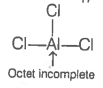Which of the following is a Lewis acid?

1. ${\mathrm{AlCl}}_{3}$

2. ${\mathrm{MgCl}}_{2}$

3. ${\mathrm{CaCl}}_{2}$

4. ${\mathrm{BaCl}}_{2}$

Hint: Lewis acids are electron-deficient, and the central atom octet is not completed.

Alkaline earth metals form ionic chloride whereas aluminium chloride is covalent. Despite of sharing electrons with chlorine, the octet of aluminium is incomplete. To complete the octet, it needs electrons and thus, acts as a Lewis acid.